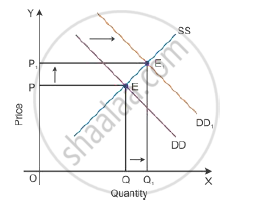Share

# Market of a Commodity is in Equilibrium. Demand for the Commodity "Increases." Explain the Chain of Effects of this Change Till the Market Again Reaches Equilibrium. Use Diagram. - CBSE (Arts) Class 12 - Economics

#### Questions

Market of a commodity is in equilibrium. Demand for the commodity "increases." Explain the chain of effects of this change till the market again reaches equilibrium. Use diagram.

Explain the chain of effects of ‘increase’ in demand of a good.

#### Solution

Consider DD to be the initial demand curve and SS to be the supply curve of the market. Market equilibrium is achieved at Point E, where the demand and supply curves intersect each other. Therefore, the equilibrium price is OP, and the equilibrium quantity demanded is OQ.When there is an increase in income of consumers, consumers would tend to spend more on normal goods than the inferior goods where the prices of all goods, tastes and preferences remain constant. There will be a shift in the demand curve towards the right to DD1 with an increase in the demand, and the supply curve SS will remain the same. This implies that at the initial price OP1, there is an excess demand of OQ to OQ1 units at the
initial price OP.

Because of an increase in the demand, there will be an increase in competition among buyers. So, they would be willing to pay more prices to obtain more units of a good in the market. Hence, there will be an increase in the market price to OP2. Now, the new market equilibrium will be at Point E1, where the new demand curve DD1 intersects the supply curve SS.

This clearly states that if the demand curve shifts towards the right, the price of the good tends to increase with an increase in the demand for a good. Thus, the direction of change in equilibrium price and quantity is the same whenever there is a shift in the demand curve.

Is there an error in this question or solution?
Solution Market of a Commodity is in Equilibrium. Demand for the Commodity "Increases." Explain the Chain of Effects of this Change Till the Market Again Reaches Equilibrium. Use Diagram. Concept: Demand Curve and Its Slope.
S